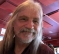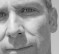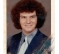## Adaptive Filter Theory: prequisites to learningStarted by 4 years ago7 replieslatest reply 4 years ago328 views

Hello!

I purchaesd Haykin (2014), "Adaptive Filter Theory," 5th ed., largely to learn about echo cancellation and other useful topics for music and audio signal processing (my favorite applications).  I have read some of his other books and was never disappointed.

Even from Chapter 1 I'm in over my head with this one.

Could somebody please recommend the prerequisite knowledge for understanding this topic and the book?

Many years ago I was working in music signal processing.  I would known what the prerequisite knowledge is and where to find it.  But I've been working in a different field for the past five years and I'm now making a big effort to sharpen up my skills.

And one project that I want to work on is to implement high quality open source echo cancellation software.

Kind regards,

Andrew

[ - ]Andrew,

I think a good place to start would be Linear Algebra.  The whole first section of that book is "Background", and all the math is based on the concept of how to sum over polynomials.  There are different names for each of the sums, and they have different properties, but the essence of all of them is same.  A z transform is sum over polynomials in time, a discrete Fourier transform is a sum over polynomials in either time or frequency, a filter is a sum over polynomials in one of those, and so on.  Once you are comfortable with summation of either finite or infinite sums, that book should be "straight forward" reading.

Systems of linear equations are solved using matrix notation.  That's also part of linear algebra.  It looks like chapter 2 uses them a lot.  I've just looked at the table of contents, but I think getting comfortable with the basic math is all you need.  You probably actually understand the ideas already - he's just using different names for things than you are used to.  Signal processing is used in a lot of fields, getting used to different terms for the same concept will help you in the long run.

Mike

[ - ]The very basics of the LMS adaptive filter are not all that difficult to understand.  Perhaps ask a question about it at the Signal Processing Stack Exchange and someone, maybe me, can try to spell out the math in as simple terms as possible.  I don't think this forum supports LaTeX math markup, does it?

You need to understand the basics of LTI systems and signals, what an FIR filter is, the basic fitting data using the least-square error criterion, and, from calculus what a function of several variables looks like and the notion of a gradient.

[ - ]$$\left(\frac{\sqrt x}{y^3}\right)$$

Inline test $$\frac{a+1}{b+1}$$

Inline test #2 $$\left(\frac{\sqrt x}{y^3}\right)$$

$$\sum_{i=0}^n i^2 = \frac{(n^2+n)(2n+1)}{6}$$

Inline test #3 $$\sum_{i=0}^n i^2 = \frac{(n^2+n)(2n+1)}{6}$$

[ - ]Stephan, your implementation of $$\LaTeX$$ here leaves a bit to be desired.  It works erratically.  especially when i edit a post that had math previously appearing.

if I have the double $, when I edit that post, one of the$ will be removed from my source.  also the inline does not always work.

let's try this:

$$\LaTeX$$

[ - ]Hi Robert,

I admit that the Mathjax implementation is not perfect and it is mostly due to the wysiwyg editor that does its own thing once in a while and mess things up.  Although not perfect and sometime frustrating, I think it is quite usable.

I did a few tests in my post right on top of yours and it went well.  I wasn't able to reproduce your double \$ this time but I know it happened to me in the past and I would love to document the issue and understand better when it is happening and why.

I will create a new post for the community to help me test Mathjax and possibly root out the bugs.

Thanks Robert.

[ - ]$$e^{i\pi}+1 =$$

$$F(\omega) = \frac{1}{2\pi} \int_{-\infty}^{+\infty} f(t) e^{-j\omega t}\ dt$$

[ - ]